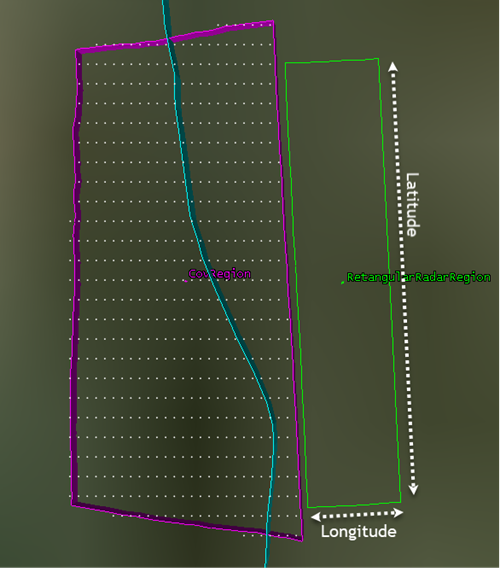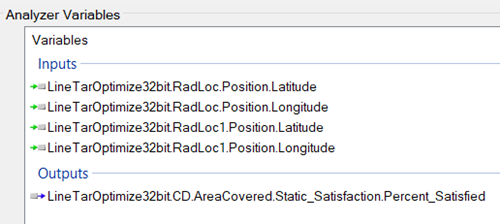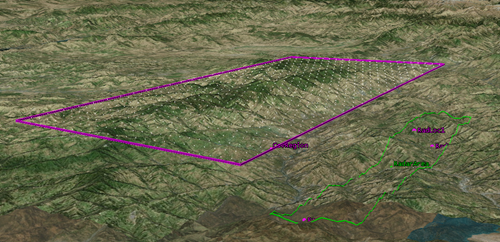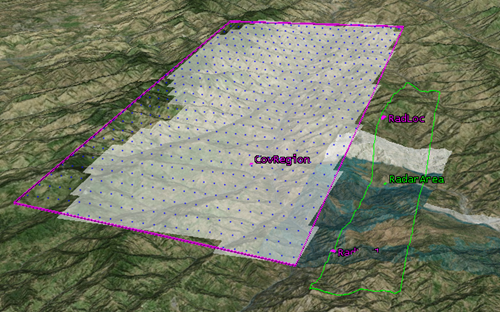Back

# Calculating the Optimal Location for Radars in a Non-rectangular Region for Persistent Surveillance

Finding the ideal locations for radars to provide persistent surveillance for a region,becomes a difficult task when the terrain is complex. Other factors that add to the complexity are height constraints, line-of-sight constraints, no-go regions etc. STK, using its gradient-based optimization algorithms, helps to locate the optimal positions for the radars. In the figure below, the magenta area shows the coverage region 3km above the terrain and the green region shows where the radars can be deployed. In this case, the radar deployment region is rectangular; hence, the optimization problem can be easily set up in Analyzer by providing the extends of latitude and longitude as the independent variable and setting the optimization objective as the percentage of Area Covered. The gradient-based algorithm can be used here. The search space for the radars will be always within the rectangular region.However, if the deployment region is non-rectangular, as shown in the figure below, and if latitude-longitude extends are used to define the search space, then there will be a totally unnecessary region (shown in red), which will be searched by the optimization algorithm.In order to restrain the search space within the green region, we propose a genetic algorithm-based optimization. To set up this in Analyzer, first create a coverage definition object on the radar deployment area target. Create a report that contains the latitude, longitude location of the points of the coverage definition. The points can be made coarser or finer as required by changing the point granularity. The genetic algorithm will search in this points cloud to determine the optimal location.

Setting up a genetic algorithm to search in these points requires a customized setup in Analyzer with macros. Here are the steps:

1. Save the points of the coverage definition as a csv file in your scenario directory.
2. Create  two (or more depending on how many radars you need to optimize) facilities in STK.
3. Set the location of those two facilities and the objective function as Analyzer variables.1. Open the Macros from Analyzer and define the two (if you are optimizing two radars) custom variables in the ‘Init’ tab.
' active=true
1. Define the following in the ‘PreRun’ Macro:
sub onPreRun()
Dim xlApp Dim xlBook Dim xlSheet Dim Count1 Dim Count2 Dim longitude1 Dim latitude1 Dim longitude2 Dim latitude2
longitude1=  xlSheet.Cells(Count1+1,2).Value
latitude1=xlSheet.Cells(Count1+1,1).Value
longitude2=  xlSheet.Cells(Count2+1,2).Value
latitude2=xlSheet.Cells(Count2+1,1).Value
end sub
1. Now select the ‘Darwin’ Optimization from the Menu of Analyzer and set RadCount1 and RadCount2 as the independent variable. The extends of these variables will be number of points in the csv file.
2. Now genetic algorithm can be run and the search space will be limited to exactly the radar deployment region.

## Case Study

In the figure below, the Magenta region shows the coverage region and the green (non-rectangular) region shows where the radars have to be deployedAn Optimization run with the Darwin Algorithm was carried out with one, two, three, and four radars. The radars have been given a maximum line-of sight visibility of 15km. The results are given below.

 Number of Radars Percentage Area Covered at Start Percentage Area Covered at End 1 Radar 10.686241947464 59.7462920172387 2 Radar 33.5271165709429 89.6514235840898 3 Radars 36.3231672981733 89.7869736960166 4 Radars 57.174744326611 91.1284347925606

It can be noted that deploying three and four radars have not provided significant improvement in coverage. Hence, it can be concluded that in this case two radars would suffice the requirement to provide optimal coverage for the magenta region.

The image below shows the maximum coverage obtained with two radars and their optimal locations.#### Systems Tool Kit (STK)

The most important thing on your desktop.

#### STK Analyzer

Perform automated trade studies with the fully integrated analysis capabilities of ModelCenter®.